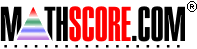Math Practice Online > free > lessons > Arizona > 2nd grade > Function Tables

Here are some tips for Function Tables, which aligns with Arizona state standards:

Function Tables

To work on this topic, you need to know your basic math facts: Fast Addition, Fast Subtraction, Fast Multiplication, and Fast Division.

A function table shows you the relationship between pairs of numbers. The relationship is defined by a rule, and this rule applies to all the pairs of numbers on the table.

You can think of the rule as a black box or machine.

 Input   → Rule →   Output input goes in the rule is applied to the input you get the output For example, 2   → Add 5 →   7

Example 1: Apply the rule

Use the given rule to fill in the missing values.
Subtract 7
Input (x)Output (y)
10
17
13
9

The rule is "Subtract 7".
This means that you need to subtract 7 from x (the input) to get y (the output).
10   →
 10 - 7
→   3
17   →
 17 - 7
→   10
13   →
 13 - 7
→   6
9   →
 9 - 7
→   2
So the answer is
Subtract 7
Input (x)Output (y)
10
17
13
9

Example 2: Apply the rule

Use the given rule to fill in the missing values.
y = x ÷ 6
Input (x)Output (y)
24
48
42
30

To apply the rule ( y = x ÷ 6), substitute the input values for x in the rule.
24   →
 24 ÷ 6
→   4
48   →
 48 ÷ 6
→   8
42   →
 42 ÷ 6
→   7
30   →
 30 ÷ 6
→   5
So the answer is
y = x ÷ 6
Input (x)Output (y)
24
48
42
30

Example 3: Find the rule

Find the rule that applies to the table.
Rule: y = x
Input (x)Output (y)
29
310
512
613
916

Look for a pattern with the input and output pairs.
Notice how every output value is greater than the input value.
This means that the rule is either addition or multiplication.

Solution 1: Look at the difference between the numbers
 The difference between 3 and 9 is 6. The difference between 4 and 10 is 6. The difference between 6 and 12 is 6. The difference between 7 and 13 is 6. The difference between 9 and 15 is 6. → For all the pairs, the difference between input and output is 6. You know that the rule is either addition or multiplication. Since the differences are all the same, the rule is addition.

The rule is y = x

Solution 2: Guess and check
Look at one pair of numbers, 3 and 9.
You know that the rule is either addition or multiplication.
Let's guess that the rule is multiplication.

How do you go from 3 → 9?
3 × 3 = 9, so maybe the rule is "multiply by 3".
Check another pair, 6 and 12.
Does 6 × 3 = 12?   No!
So, multiplication is not right.
Now, let's guess that the rule is addition.
How do you go from 3 → 9?
3 + 6 = 9, so maybe the rule is "add by 6".
Check another pair, 6 and 12.
Does 6 + 6 = 12?   Yes!
Check another pair, 9 and 15.
Does 9 + 6 = 15?   Yes!
(You can check all the other pairs to make sure.)
So, addition is right.
The rule is y = x

Example 4: Find the rule

Find the rule that applies to the table.
Rule: y = x
Input (x)Output (y)
81
243
405
648
729

Look for a pattern with the input and output pairs.
Notice how every output value is less than the input value.
This means that the rule is either subtraction or division.

Solution 1: Look at the difference between the numbers
 The difference between 8 and 1 is 7. The difference between 24 and 3 is 21. The difference between 40 and 5 is 35. The difference between 64 and 8 is 56. The difference between 72 and 9 is 63. → The differences between the input and output pairs are different. You know that the rule is either subtraction or division. Since the differences are all different, the rule is division.

How do you go from 8 → 1?   8 ÷ 8 = 1
How do you go from 24 → 3?   24 ÷ 8 = 3
So the rule is division by 8.

The rule is y = x

Solution 2: Guess and check
Look at one pair of numbers, 8 and 1.
You know that the rule is either subtraction or division.
Let's guess that the rule is subtraction.

How do you go from 8 → 1?
8 - 7 = 1, so maybe the rule is "subtract 7".
Check another pair, 24 and 3.
Does 24 - 7 = 3?   No!
So, subtraction is not right.
Now, let's uess that the rule is division.
How do you go from 8 → 1?
8 ÷ 8 = 1, so maybe the rule is "divide by 6".
Check another pair, 24 and 3.
Does 24 ÷ 8 = 3?   Yes!
Check another pair, 40 and 5.
Does 40 ÷ 8 = 5?   Yes!
(You can check all the other pairs to make sure.)
So, division is right.
The rule is y = x

Copyright Accurate Learning Systems Corporation 2008.
MathScore is a registered trademark.